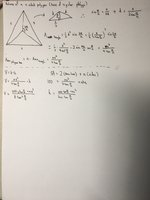# Investigation of a n-sided prism.

#### dylanbui

##### New member
Currently I am working on a project with optimization of prisms with a regular polygon base. It is similar to the investigation detailed here:https://ibmathsresources.com/2017/05/21/optimization-of-area-an-investigation/. In my project, a surface area of 100 is , and I'm trying to find the maximum volume a n-sided prisms will have. Anyways the problem is I end up with only two equation with three variables, and I am trying to find the optimal volume.

Sorry if this explanation is confusing, the attachment should explain it better.#### Dr.Peterson

##### Elite Member
You haven't quite stated what your goal is. Is it, first, to find the largest possible volume for a regular n-gonal prism with surface area 100? I see only two variables there (s and h, taking n as fixed), so two equations should be all you need. It looks like you've expressed V as a function of s alone; find the maximum.

Then you can consider what happens when n changes.

#### LCKurtz

##### Full Member
To simplify a bit what you have written, let's call $$\displaystyle A_b$$ the area of the base, $$\displaystyle S$$ the total surface area, $$\displaystyle n$$ the number of sides, $$\displaystyle s$$ the length of a side, and $$\displaystyle h$$ the height. So you have $$\displaystyle A_bh = 100$$ and $$\displaystyle S=2A_b + nsh$$. You can eliminate the $$\displaystyle A_b$$ between these two and write $$\displaystyle S = \frac {200} h + nsh$$. But $$\displaystyle n$$ and $$\displaystyle s$$ depend on each other, and both depend on the radius, which you have called $$\displaystyle d$$, of the circumscribing circle. Is a fixed radius given or is it allowed to vary? The relation between $$\displaystyle d$$ and $$\displaystyle s$$ is $$\displaystyle d = \frac{s}{2\sin\frac \pi n}$$.

#### dylanbui

##### New member
You haven't quite stated what your goal is. Is it, first, to find the largest possible volume for a regular n-gonal prism with surface area 100? I see only two variables there (s and h, taking n as fixed), so two equations should be all you need. It looks like you've expressed V as a function of s alone; find the maximum.

Then you can consider what happens when n changes.
Yes, that is my goal. I am trying to find an equation that gives the optimal height and side for a given n.
I am a bit confused about n being fixed.
I have expressed V as a function of s and n.

#### dylanbui

##### New member
To simplify a bit what you have written, let's call $$\displaystyle A_b$$ the area of the base, $$\displaystyle S$$ the total surface area, $$\displaystyle n$$ the number of sides, $$\displaystyle s$$ the length of a side, and $$\displaystyle h$$ the height. So you have $$\displaystyle A_bh = 100$$ and $$\displaystyle S=2A_b + nsh$$. You can eliminate the $$\displaystyle A_b$$ between these two and write $$\displaystyle S = \frac {200} h + nsh$$. But $$\displaystyle n$$ and $$\displaystyle s$$ depend on each other, and both depend on the radius, which you have called $$\displaystyle d$$, of the circumscribing circle. Is a fixed radius given or is it allowed to vary? The relation between $$\displaystyle d$$ and $$\displaystyle s$$ is $$\displaystyle d = \frac{s}{2\sin\frac \pi n}$$.
I am not sure what you mean about being inscribed in a circle, but d can vary based on n and s.
It is the surface area that equals 100, so S=100

#### Dr.Peterson

##### Elite Member
Yes, that is my goal. I am trying to find an equation that gives the optimal height and side for a given n.
I am a bit confused about n being fixed.
I have expressed V as a function of s and n.
Find the optimal volume with n=3, then with n=4, and so on. Most of the work will be done in the general case (leaving n as a variable, but treating it as a constant).